Cleave Books
Quick Puzzles ~ Set 2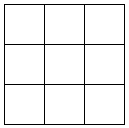1. Draw a 3 by 3 grid like that shown and colour the squares either
Red , White , or Blue
so that
each Red touches a White
eachWhite touches a Blue
each Blue touches a Red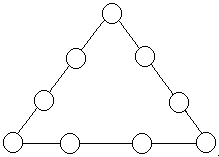2. Make a copy of a triangle like that shown with four circles along each edge. Use the numbers 1 to 9. putting one in each circle so that the four numbers along each edge add up to the same total. Find two different ways of doing this.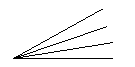3. How many acute angles can be found in this drawing?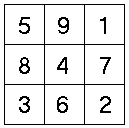4. On this diagram you may start at any square and move up or down or across (but NOT diagonally) into the next square. No square may be used twice. The digits in each square are written down in the order they are used.
What is the largest number that can be made?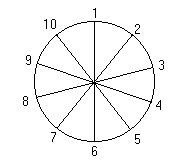5. Copy the diagram on the right but re-arrange the numbers so that any pair which are side by side, add up to the same total as the pair opposite. At the moment it is only true for
1+10 = 5+6.6. The French Flag (known as the tricolour) is coloured
Red White and Blue
in the way shown. Using the same three colours, how many different flags would it be possible to make?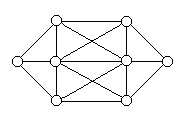7. Copy out the diagram like that on the right. Use the numbers 1 to 8, putting one in each circle, in such a way that no two circles which are connected by a single straight line, contain two consecutive numbers.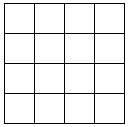8. Copy this grid and colour it with
4 blue squares
3 red squares
3 green squares
3 yellow squares
3 white squares .
so that NO two squares of the same colour are in line, either vertically, horizontally or diagonally.9. Draw out a 3 by 3 grid like that shown. Place the numbers -
2 2 2 3 3 3 4 4 4
in it so that, when any line of three numbers is added up in any direction (including diagonally), the total is always 9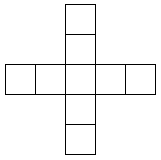10.Arrange the numbers 1 to 9, using each one only once, placing only one number in each cell so that the totals in both directions
( up and down and across) are the same. How may different ways are there of doing this?